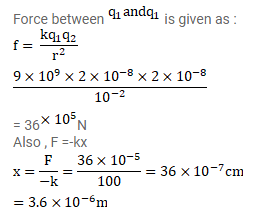# Two identically charged particles are fastened to the two rnds ofQuestion:

Two identically charged particles are fastened to the two rnds of a spring of spring constant . $100 \mathrm{~N} / \mathrm{m}$ and natural length $10 \mathrm{~cm}$. the system rests on a smooth horizontal table. If the charge on each particle is $2.0 \times 10^{-8} \mathrm{C}$, find the extension in the length of the spring. Assume that the extension is small as compared to the natural length. Justify this assumption after you solve the problem.

Solution: